# Receptive Fields in Convolutional Neural Networks

In this article I will very briefly present convolutional neural networks, their two padding strategies, and what is their receptive field (RF). In addition I review three possible strategies to make our RF larger and their trade-offs. Finally, we will see how dilation applies to transposed convolutions (I described them in my previous post), and what is the RF in this case. Everything is explained for the 1D case, but extending it to images (2D), video (3D), or even higher dimensional signals is straightforward, just replicate kernel dimensions symmetrically (not channels, just time/space dimensions).

# Convolutional neural networks (1D)

Let’s first recap what happens in a 1D convolutional layer, with a single neuron, 3 weights `w = [w1, w2, w3]` and an input signal `x = [x1, x2, x3]` with one channel:

Actually we can see how a single convolutional neuron is not different from a feed forward neuron if we match the input length with the amount of kernel connections (3). The difference with a feed forward neuron comes when we have an input length `L `larger than the kernel length `N`, i.e. `L > N :`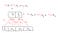Convolutional neuron sliding through the input. L = 4 and N = 3.

We can now see that the convolution outputs two elements, sliding its window of length 3 over the inputs, in a one by one position stride. Moreover, if we put a spoon of padding in our recipe we can match the output length we want too.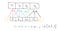Convolution with padding. Now the convolution operation has same output length as real input by injecting some fake cells that are null. Colors denote same weights, but clarify separate kernel operations.

So far we can already build two kinds of convolutional layers, and both will have the same notion of receptive field (I will reach the receptive field concept very soon!): (1) Vanilla convolutions, which operate as we see in previous figure, symmetrically with respect to a central point; and (2) causal convolutions, which operate seeing only past-present to predict present, which I depict in next figure bellow.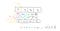Causal convolution left-to-right directional. Yellow triangle shows past-present dependency on x data to predict next y points.

This processes data without looking into future of input to predict the output. This is the core structure of the famous WaveNet .

Now what is the receptive field of the neuron? Well it is actually the yellow triangle we see in the figure above: the amount of context that a neuron sees in the input to predict its output. So a single layer has a receptive field `R = len(w) = N` . But what happens when we stack layers? It gets larger of course.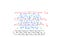Green arrows show Layer 3 neuron’s receptive field, spanning to 7 samples with a stack of 3 layers of size 3.

# How to make really large receptive fields?

Now we know what is the receptive field of a certain neuron inside a convolutional network. But normally we want a large receptive field after a deep composition. We have seen that stacking is a possibility, but receptive field grows slowly with depth with fixed little kernels. The three ways in which we can increase the RF are:

1. Make larger kernel sizes
2. Use higher stride or pooling
3. Using dilation factors in the kernels

The first option can be seen by looking at figure above and picturing a larger kernel (e.g. 5, 7, 11, etc.). Second option is pictured in figure below, where whenever we have a reduction in signal length in the `out_len/in_len` ratio (e.g. `0.5` ), receptive field increases substantially with a fixed kernel size.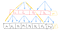Example conv1D with 2 layers, kernel size 3 and stride 2

In the figure above we can see how stride is now 2 instead of 1(no overlap in blue arrows). This is already giving us a signal which is halved in temporal resolution, which made us achieve a receptive field of 7 with 1 less layer. This is a very little example of course, but imagine the exponential increase in receptive field when we have 2, 3 or more decimation layers with factor 2 (or more). The growth is comparable to that of our third method, dilated convolutions.

The figure below shows the dilation method, where dilation stands for “crossing-out” `d-1` elements between two kernel weights. The value `d` is called dilation factor. In the case below, we have a kernel of size 3 and dilation 1 in the first layer. This ensures all samples in the input sequence are seen in the first hidden features, and from that point onwards there will be processing blanks in order to increase the RF in a quick manner. So second layer dilation factor is `d=2` which already gives us a virtual kernel size of 5 in that layer, although we estimate the parameters of kernels of length 3! With dilation `d=4` we have a virtual kernel size of 9, and we can keep increasing it in upper layers of course.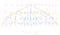Dilated convolutional network of 3 layers, kernel size 3 and dilation factors d=1, d=2 and d=4 in increasing layer index.

We have these (2) and (3) mechanisms to increase our receptive field at a fixed size of parameters from our original network, which were kernels of size 3. What is the trade-off of these (2) and (3) methods though?

# Beware with memory and processing time

Method (2) is a decimation, so each decimated layer will be faster to process (convolutional kernel strides less times with less time-steps). However, it will only be helpful when you want to compress your data for classification or to build an auto-encoder like architecture (at least normally). Another advantage of this method is memory saving, because less time resolution means less data points to store as intermediate feature map activations through your network!

Method (3) does not decimate, so you respect signal time-resolution and extract some features at every stage, but it come at the price of higher memory consumption and lower speed. With highly performant matrix computing units like GPUs speed is not dramatically damaged in many cases (whenever we don’t have an auto-regressive model), but memory can be! In any case, this perfectly fits models like the WaveNet (van den Oord et al. 2016) or parallel WaveNet (van den Oord et al. 2017).

# Last but not least: how does a deconvolution look like when we give it dilation?

Perhaps some of you readers have noticed the possibility to use dilation in the so called transposed convolutions (or deconvolutions). For instance, in the PyTorch ConvTranspose1d documentation there is a dilation argument:

In my previous post I described, pictured and exemplified with code what deconvolutions are. There you will see it is a reverse-convolution (roughly speaking). Well we have an interesting fact: we choose how our up-sample factor is created. Either we use a continuous sliding filter by using a `stride > 1` (remember, with kernel width `kwidth >= stride` ), or we let window slide with `stride = 1` and use the dilation factor `d > 1` to achieve the output interpolation we require. The figure below depicts the two possibilities. We could say that in both cases each kernel has a RF of 2, so that every `y` sample is responsible of generating 2 `x` samples.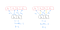Left: typical well-known interpolation with a “deconvolution” of continuous non-overlapping strided kernel of width 2, stride 2 and dilation 1. Right: interleaved implementation with the same kernel of width 2, stride 1 and dilation 2. Yellow crossed out arrows depict the blanks in kernel connections given the dilation factors.

Is it a good idea to use an interleaved deconvolution? It depends on your application, I do not have a clear answer to this though (yet)! What is the key message here though? There is no unique way to achieve an encoder/decoder effect. There are many factors to design a convolutional/deconvolutional neural network, so that the dimensionality we get is the desired one, and so that every neuron in the deepest part of the structure has access to enough input context to make decisions. These factors include not only kernel width and paddings, but also dilations and strides.

# Summary

We have reviewed what is a convolutional neural network, understanding it as a stack of convolutional layers. We have also briefly seen the simple padding trick that brings us causal convolutional neural networks. Then we showed the definition of a neuron’s receptive field (RF), and how we can manipulate network parameters to make it larger. Three main strategies are available: (1) modifying kernel size, (2) building decimating structures like strided convolutions (max/average pooling would work too), and (3) using dilated convolutions. We have also seen how dilation is interpreted in the transposed convolutions, and how they give us new ways to achieve interpolation factors with an interleaved decoding strategy.

PhD from UPC Barcelona. Telecom Engineer and Deep Learner. Generative models rock. I DO learn for fun.

## More from Santi Pdp

PhD from UPC Barcelona. Telecom Engineer and Deep Learner. Generative models rock. I DO learn for fun.